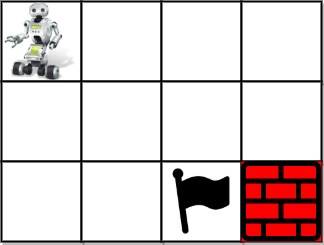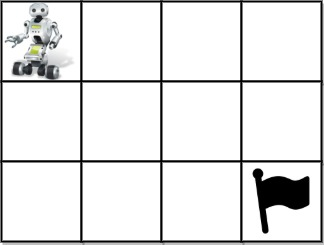# Unique Paths III LeetCode Solution

Difficulty Level Hard
LimeBikeViews 1621

## Problem Statement:

Unique Paths III LeetCode Solution: You are given an `m x n` integer array `grid` where `grid[i][j]` could be:

• `1` representing the starting square. There is exactly one starting square.
• `2` representing the ending square. There is exactly one ending square.
• `0` representing empty squares we can walk over.
• `-1` representing obstacles that we cannot walk over.

Return the number of 4-directional walks from the starting square to the ending square, that walk over every non-obstacle square exactly once.

## Examples:

Example 1:Input:

``` grid = [[1,0,0,0],[0,0,0,0],[0,0,2,-1]]
```

Output:

``` 2
```

Explanation:

``` We have the following two paths:
1. (0,0),(0,1),(0,2),(0,3),(1,3),(1,2),(1,1),(1,0),(2,0),(2,1),(2,2)
2. (0,0),(1,0),(2,0),(2,1),(1,1),(0,1),(0,2),(0,3),(1,3),(1,2),(2,2)
```

Example 2:Input:

``` grid = [[1,0,0,0],[0,0,0,0],[0,0,0,2]]
```

Output:

``` 4
```

Explanation:

``` We have the following four paths:
1. (0,0),(0,1),(0,2),(0,3),(1,3),(1,2),(1,1),(1,0),(2,0),(2,1),(2,2),(2,3)
2. (0,0),(0,1),(1,1),(1,0),(2,0),(2,1),(2,2),(1,2),(0,2),(0,3),(1,3),(2,3)
3. (0,0),(1,0),(2,0),(2,1),(2,2),(1,2),(1,1),(0,1),(0,2),(0,3),(1,3),(2,3)
4. (0,0),(1,0),(2,0),(2,1),(1,1),(0,1),(0,2),(0,3),(1,3),(1,2),(2,2),(2,3)
```

Example 3:Input:

``` grid = [[0,1],[2,0]]
```

Output:

``` 0
```

Explanation:

```There is no path that walks over every empty square exactly once.
Note that the starting and ending square can be anywhere in the grid.```

## Approach:

### Idea:

The problem can be solved with the concept of backtracking and DFS. First, we will count the total number of non-obstacle cells. Starting from the initial cell we will do a DFS. Once we reach the destination cell after covering all the non-obstacle cells we will increment our count, considering this path valid. We will use a visited array to mark all the visited cells.

If you look carefully at your problem constraints: `m*n <= 20`, where `m` and `n` your grid sizes, we can guess, that we need to use dfs in this problem: that is try to check all possible options. So, we need to do several steps:

1. Find our starting point: traverse all grid and find cell with value `1`. Also count number of cells we need to visit, I denoted them `empty`.
2. Now, use recursive `dfs(x,y, rest)` function, where `x` and `y` are current coordinates in our cell and `rest` is how many empty cells we need to traverse.
2.1 First we check if we can move to the current cell: if it is outside our grid and it is already visited or have obstacle, we return
2.2 If current cell is end cell and we already visited all cells, we add `1` to `self.ans`.
2.3 Define list of neighbours and for each of them run our `dfs`: mark visited cell with `-2` and then mark it back when we go outside recursion. It is important to do this, because in python `grid` is global variable and we need to change it back.
3. FInally, we just run `dfs(sx, sy, empty - 1)`, where `(sx, sy)` is coordinates of starting cell and return `self.ans`.

### Code:

Unique Paths III C++ Solution:

```class Solution {
public:
int cells = 0;
int n,m;
int ans = 0;
vector<vector<int>> dirs = {{0,1},{1,0},{0,-1},{-1,0}};

void helper(int i,int j,int cnt,vector<vector<int>>& grid,vector<vector<int>>& vis){
if(grid[i][j] == 2 and cnt == cells){
ans++;
return;
}
for(auto it:dirs){
int x = i + it;
int y = j + it;

if(x>=0 and y>=0 and x<n and y<m and grid[x][y] != -1 and vis[x][y] == 0){
vis[x][y] = 1;
helper(x,y,cnt+1,grid,vis);
vis[x][y] = 0;
}
}
}

int uniquePathsIII(vector<vector<int>>& grid) {
n = grid.size();
m = grid.size();
vector<vector<int>> vis(n,vector<int>(m,0));
int x = 0;
int y = 0;
for(int i=0;i<n;i++){
for(int j=0;j<m;j++){
if(grid[i][j] == 1){
x = i;
y = j;
}
if(grid[i][j] == 0)
cells++;
}
}
vis[x][y] = 1;
cells++;
helper(x,y,0,grid,vis);
return ans;
}
};```

Translate »